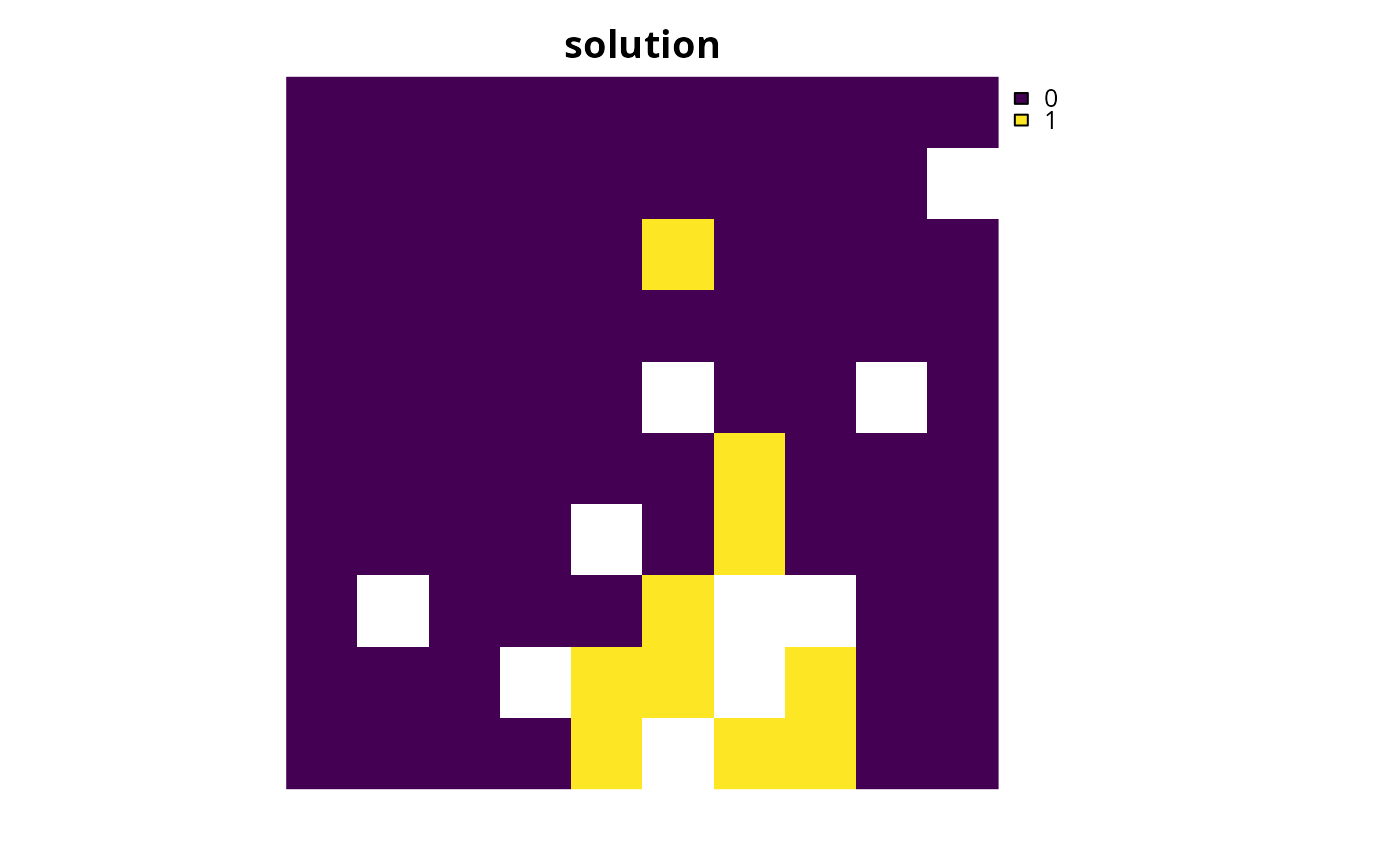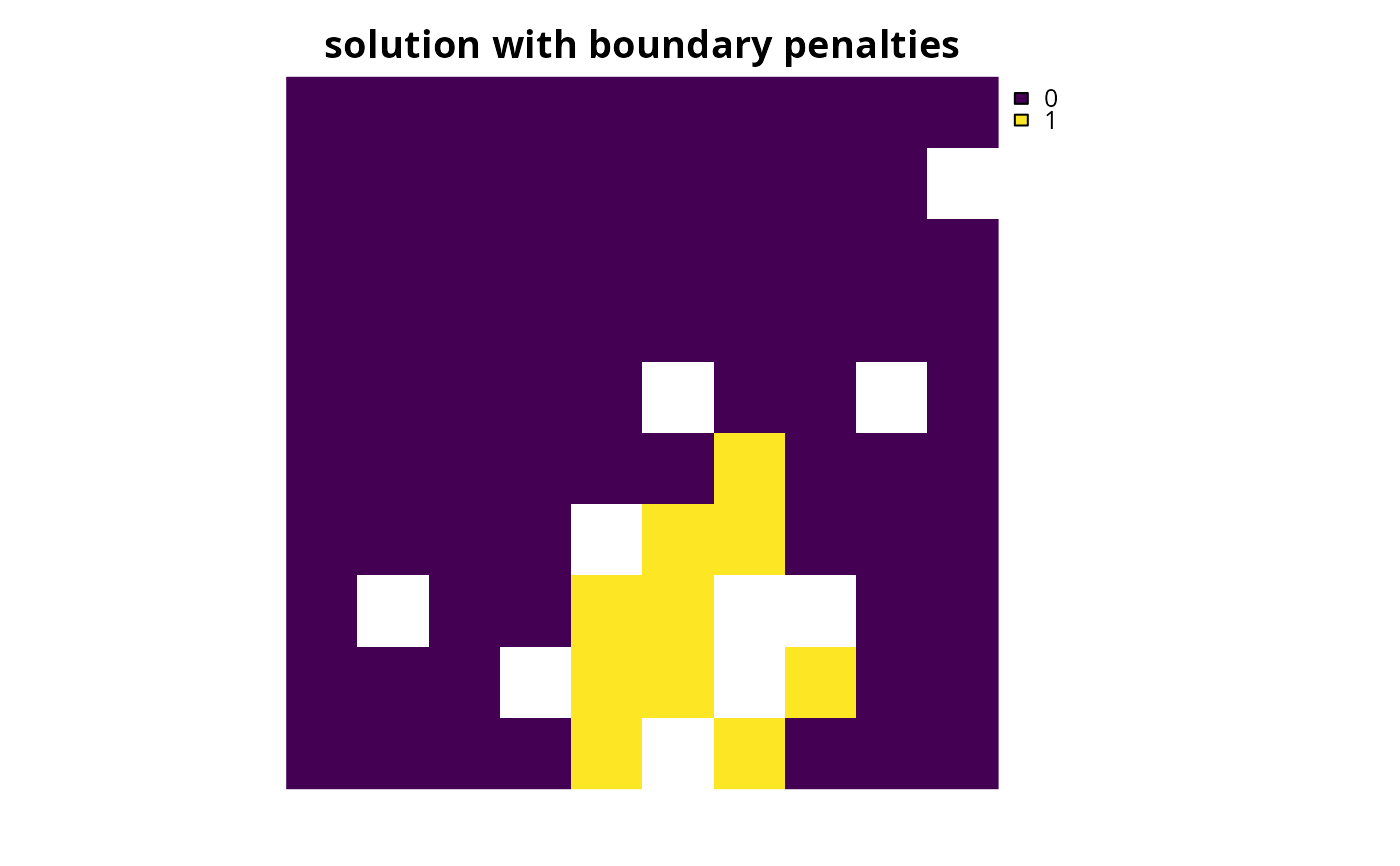Specify that the CBC (COIN-OR branch and cut) software should be used to solve a conservation planning problem (Forrest & Lougee-Heimer 2005). This function can also be used to customize the behavior of the solver. It requires the rcbc package to be installed (only available on GitHub, see below for installation instructions).

add_cbc_solver(
x,
gap = 0.1,
time_limit = .Machine$integer.max, presolve = TRUE, threads = 1, first_feasible = FALSE, start_solution = NULL, verbose = TRUE ) ## Arguments x problem() object. gap numeric gap to optimality. This gap is relative and expresses the acceptable deviance from the optimal objective. For example, a value of 0.01 will result in the solver stopping when it has found a solution within 1% of optimality. Additionally, a value of 0 will result in the solver stopping when it has found an optimal solution. The default value is 0.1 (i.e., 10% from optimality). time_limit numeric time limit (seconds) for generating solutions. The solver will return the current best solution when this time limit is exceeded. The default value is the largest integer value (i.e., .Machine$integer.max), effectively meaning that solver will keep running until a solution within the optimality gap is found.

presolve

logical attempt to simplify the problem before solving it? Defaults to TRUE.

integer number of threads to use for the optimization algorithm. The default value is 1.

first_feasible

logical should the first feasible solution be be returned? If first_feasible is set to TRUE, the solver will return the first solution it encounters that meets all the constraints, regardless of solution quality. Note that the first feasible solution is not an arbitrary solution, rather it is derived from the relaxed solution, and is therefore often reasonably close to optimality. Defaults to FALSE.

start_solution

NULL or object containing the starting solution for the solver. This is can be useful because specifying a starting solution can speed up the optimization process. Defaults to NULL such that no starting solution is used. To specify a starting solution, the argument to start_solution should be in the same format as the planning units (i.e., a NULL, numeric, matrix, data.frame, terra::rast(), or sf::sf() object). See the Start solution format section for more information.

verbose

logical should information be printed while solving optimization problems? Defaults to TRUE.

## Value

An updated problem() object with the solver added to it.

## Details

CBC is an open-source mixed integer programming solver that is part of the Computational Infrastructure for Operations Research (COIN-OR) project. This solver seems to have much better performance than the other open-source solvers (i.e., add_highs_solver(), add_rsymphony_solver(), add_lpsymphony_solver()) (see the Solver benchmarks vignette for details). As such, it is strongly recommended to use this solver if the Gurobi and IBM CPLEX solvers are not available.

## Installation

The rcbc package is required to use this solver. Since the rcbc package is not available on the the Comprehensive R Archive Network (CRAN), it must be installed from its GitHub repository. To install the rcbc package, please use the following code:

if (!require(remotes)) install.packages("remotes")
remotes::install_github("dirkschumacher/rcbc")

Note that you may also need to install several dependencies -- such as the Rtools software or system libraries -- prior to installing the rcbc package. For further details on installing this package, please consult the online package documentation.

## Start solution format

Broadly speaking, the argument to start_solution must be in the same format as the planning unit data in the argument to x. Further details on the correct format are listed separately for each of the different planning unit data formats:

x has numeric planning units

The argument to start_solution must be a numeric vector with each element corresponding to a different planning unit. It should have the same number of planning units as those in the argument to x. Additionally, any planning units missing cost (NA) values should also have missing (NA) values in the argument to start_solution.

x has matrix planning units

The argument to start_solution must be a matrix vector with each row corresponding to a different planning unit, and each column correspond to a different management zone. It should have the same number of planning units and zones as those in the argument to x. Additionally, any planning units missing cost (NA) values for a particular zone should also have a missing (NA) values in the argument to start_solution.

x has terra::rast() planning units

The argument to start_solution be a terra::rast() object where different grid cells (pixels) correspond to different planning units and layers correspond to a different management zones. It should have the same dimensionality (rows, columns, layers), resolution, extent, and coordinate reference system as the planning units in the argument to x. Additionally, any planning units missing cost (NA) values for a particular zone should also have missing (NA) values in the argument to start_solution.

x has data.frame planning units

The argument to start_solution must be a data.frame with each column corresponding to a different zone, each row corresponding to a different planning unit, and cell values corresponding to the solution value. This means that if a data.frame object containing the solution also contains additional columns, then these columns will need to be subsetted prior to using this function (see below for example with sf::sf() data). Additionally, any planning units missing cost (NA) values for a particular zone should also have missing (NA) values in the argument to start_solution.

x has sf::sf() planning units

The argument to start_solution must be a sf::sf() object with each column corresponding to a different zone, each row corresponding to a different planning unit, and cell values corresponding to the solution value. This means that if the sf::sf() object containing the solution also contains additional columns, then these columns will need to be subsetted prior to using this function (see below for example). Additionally, the argument to start_solution must also have the same coordinate reference system as the planning unit data. Furthermore, any planning units missing cost (NA) values for a particular zone should also have missing (NA) values in the argument to start_solution.

Other solvers: add_cplex_solver(), add_default_solver(), add_gurobi_solver(), add_highs_solver(), add_lsymphony_solver, add_rsymphony_solver()

## Examples

# \dontrun{
sim_pu_raster <- get_sim_pu_raster()
sim_features <- get_sim_features()

# create problem
p1 <-
problem(sim_pu_raster, sim_features) %>%
add_cbc_solver(gap = 0, verbose = FALSE)

# generate solution %>%
s1 <- solve(p1)

# plot solution
plot(s1, main = "solution", axes = FALSE)# create a similar problem with boundary length penalties and
# specify the solution from the previous run as a starting solution
p2 <-
problem(sim_pu_raster, sim_features) %>%